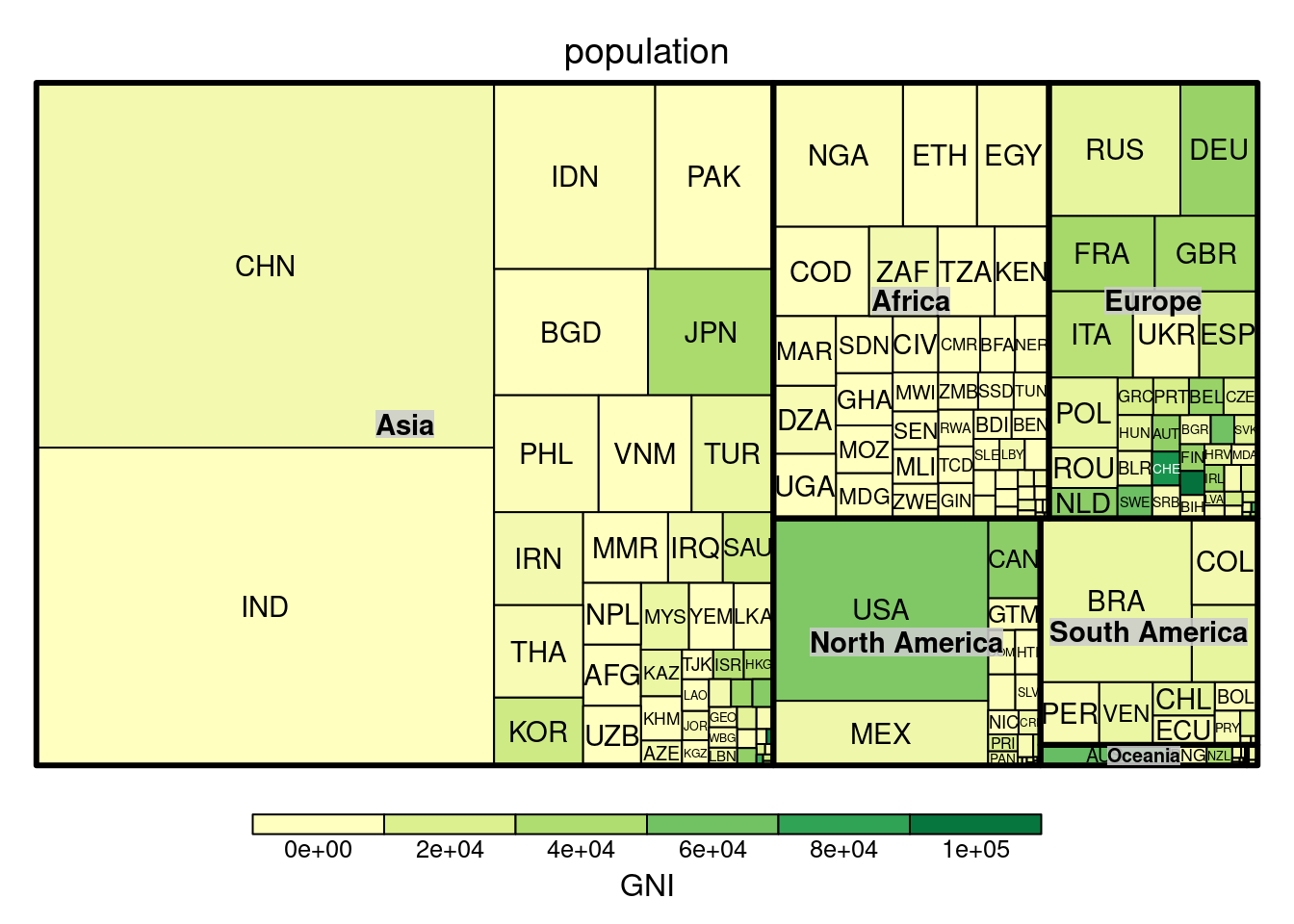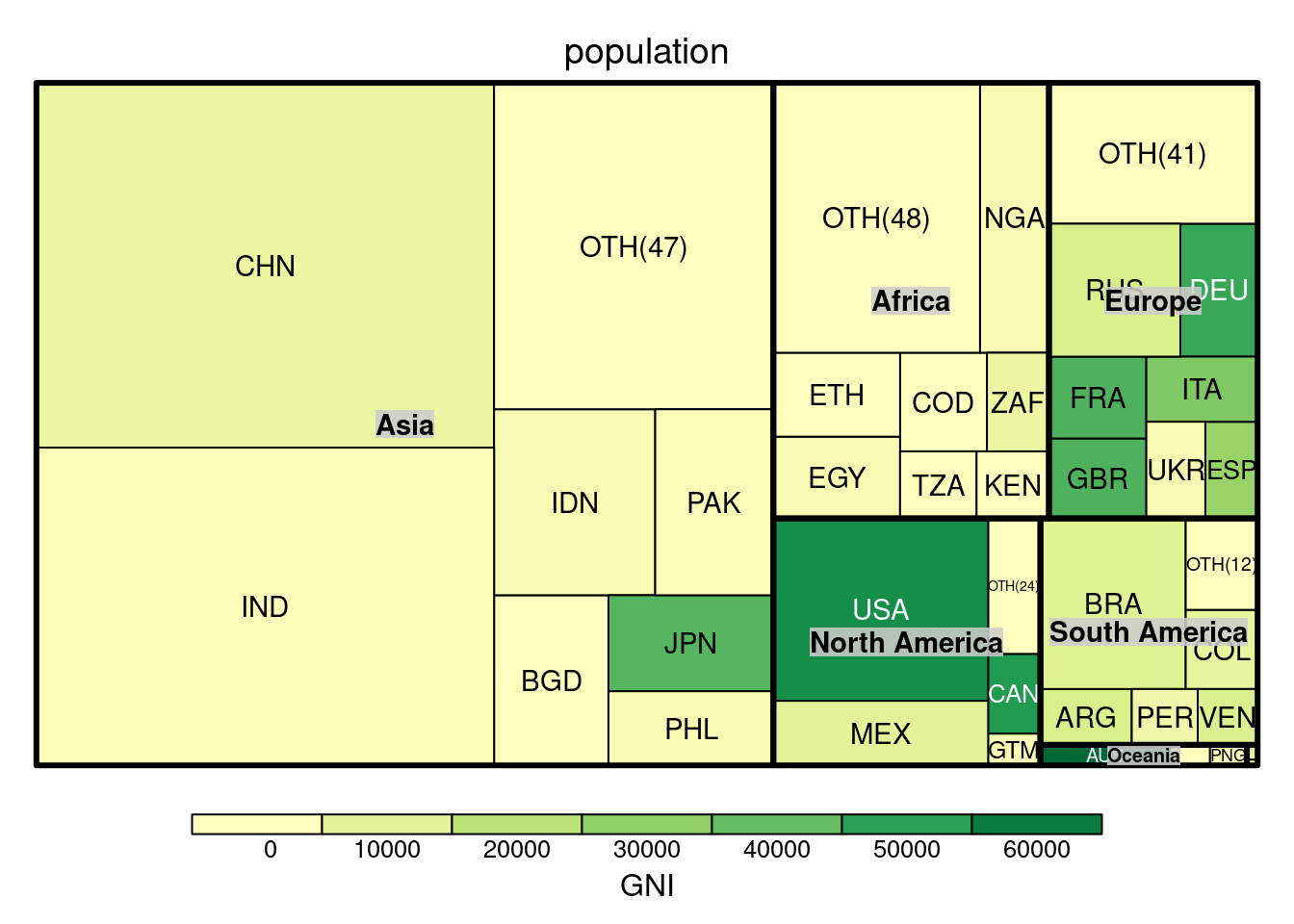# Introduction

This vignette gives you a quick introduction to data.tree applications. We took care to keep the examples simple enough so non-specialists can follow them. The price for this is, obviously, that the examples are often simple compared to real-life applications.

If you are using data.tree for things not listed here, and if you believe this is of general interest, then please do drop us a note, so we can include your application in a future version of this vignette.

# World PopulationTreeMap (visualization)

This example is inspired by the examples of the treemap package.

You’ll learn how to

• convert a data.frame to a data.tree structure
• navigate a tree and locate specific nodes
• use `Aggregate` and `Cumulate`
• manipulate an existing tree, e.g. by using the `Prune` method

## Original Example, to be improved

The original example visualizes the world population as a tree map.

``````library(treemap)
data(GNI2014)
treemap(GNI2014,
index=c("continent", "iso3"),
vSize="population",
vColor="GNI",
type="value")``````As there are many countries, the chart gets clustered with many very small boxes. In this example, we will limit the number of countries and sum the remaining population in a catch-all country called “Other”.

We use data.tree to do this aggregation.

## Convert from data.frame

First, let’s convert the population data into a data.tree structure:

``````library(data.tree)
GNI2014\$continent <- as.character(GNI2014\$continent)
GNI2014\$pathString <- paste("world", GNI2014\$continent, GNI2014\$country, sep = "/")
tree <- as.Node(GNI2014[,])
print(tree, pruneMethod = "dist", limit = 20)``````
``````##                        levelName
## 1  world
## 2   ¦--North America
## 3   ¦   ¦--Bermuda
## 4   ¦   ¦--United States
## 5   ¦   °--... 22 nodes w/ 0 sub
## 6   ¦--Europe
## 7   ¦   ¦--Norway
## 8   ¦   ¦--Switzerland
## 9   ¦   °--... 39 nodes w/ 0 sub
## 10  ¦--Asia
## 11  ¦   ¦--Qatar
## 12  ¦   ¦--Macao SAR, China
## 13  ¦   °--... 45 nodes w/ 0 sub
## 14  ¦--Oceania
## 15  ¦   ¦--Australia
## 16  ¦   ¦--New Zealand
## 17  ¦   °--... 11 nodes w/ 0 sub
## 18  ¦--South America
## 19  ¦   ¦--Uruguay
## 20  ¦   ¦--Chile
## 21  ¦   °--... 10 nodes w/ 0 sub
## 22  ¦--Seven seas (open ocean)
## 23  ¦   ¦--Seychelles
## 24  ¦   ¦--Mauritius
## 25  ¦   °--... 1 nodes w/ 0 sub
## 26  °--Africa
## 27      °--... 48 nodes w/ 0 sub``````

We can also navigate the tree to find the population of a specific country. Luckily, RStudio is quite helpful with its code completion (use `CTRL + SPACE`):

``tree\$Europe\$Switzerland\$population``
``##  7604467``

Or, we can look at a sub-tree:

``````northAm <- tree\$`North America`
Sort(northAm, "GNI", decreasing = TRUE)
print(northAm, "iso3", "population", "GNI", limit = 12)``````
``````##                    levelName iso3 population    GNI
## 1  North America                          NA     NA
## 2   ¦--Bermuda                BMU      67837 106140
## 3   ¦--United States          USA  313973000  55200
## 4   ¦--Canada                 CAN   33487208  51630
## 5   ¦--Bahamas, The           BHS     309156  20980
## 6   ¦--Trinidad and Tobago    TTO    1310000  20070
## 7   ¦--Puerto Rico            PRI    3971020  19310
## 8   ¦--Barbados               BRB     284589  15310
## 9   ¦--St. Kitts and Nevis    KNA      40131  14920
## 10  ¦--Antigua and Barbuda    ATG      85632  13300
## 11  ¦--Panama                 PAN    3360474  11130
## 12  °--... 14 nodes w/ 0 sub              NA     NA``````

Or, we can find out what is the country with the largest GNI:

``````maxGNI <- Aggregate(tree, "GNI", max)
#same thing, in a more traditional way:
maxGNI <- max(sapply(tree\$leaves, function(x) x\$GNI))

tree\$Get("name", filterFun = function(x) x\$isLeaf && x\$GNI == maxGNI)``````
``````##   Bermuda
## "Bermuda"``````

## Aggregate and Cumulate

We aggregate the population. For non-leaves, this will recursively iterate through children, and cache the result in the `population` field.

``````tree\$Do(function(x) {
x\$population <- Aggregate(node = x,
attribute = "population",
aggFun = sum)
},
traversal = "post-order")``````

Next, we sort each node by population:

``Sort(tree, attribute = "population", decreasing = TRUE, recursive = TRUE)``

Finally, we cumulate among siblings, and store the running sum in an attribute called `cumPop`:

``tree\$Do(function(x) x\$cumPop <- Cumulate(x, "population", sum))``

The tree now looks like this:

``print(tree, "population", "cumPop", pruneMethod = "dist", limit = 20)``
``````##                        levelName population     cumPop
## 1  world                         6683146875 6683146875
## 2   ¦--Asia                      4033277009 4033277009
## 3   ¦   ¦--China                 1338612970 1338612970
## 4   ¦   ¦--India                 1166079220 2504692190
## 5   ¦   °--... 45 nodes w/ 0 sub         NA         NA
## 6   ¦--Africa                     962382035 4995659044
## 7   ¦   ¦--Nigeria                149229090  149229090
## 8   ¦   ¦--Ethiopia                85237338  234466428
## 9   ¦   °--... 46 nodes w/ 0 sub         NA         NA
## 10  ¦--Europe                     728669949 5724328993
## 11  ¦   ¦--Russian Federation     140041247  140041247
## 12  ¦   ¦--Germany                 82329758  222371005
## 13  ¦   °--... 39 nodes w/ 0 sub         NA         NA
## 14  ¦--North America              528748158 6253077151
## 15  ¦   ¦--United States          313973000  313973000
## 16  ¦   ¦--Mexico                 111211789  425184789
## 17  ¦   °--... 22 nodes w/ 0 sub         NA         NA
## 18  ¦--South America              394352338 6647429489
## 19  ¦   ¦--Brazil                 198739269  198739269
## 20  ¦   ¦--Colombia                45644023  244383292
## 21  ¦   °--... 10 nodes w/ 0 sub         NA         NA
## 22  ¦--Oceania                     33949312 6681378801
## 23  ¦   ¦--Australia               21262641   21262641
## 24  ¦   ¦--Papua New Guinea         6057263   27319904
## 25  ¦   °--... 11 nodes w/ 0 sub         NA         NA
## 26  °--Seven seas (open ocean)      1768074 6683146875
## 27      °--... 3 nodes w/ 0 sub          NA         NA``````

## Prune

The previous steps were done to define our threshold: big countries should be displayed, while small ones should be grouped together. This lets us define a pruning function that will allow a maximum of 7 countries per continent, and that will prune all countries making up less than 90% of a continent’s population.

We would like to store the original number of countries for further use:

``tree\$Do(function(x) x\$origCount <- x\$count)``

We are now ready to prune. This is done by defining a pruning function, returning ‘FALSE’ for all countries that should be combined:

``````myPruneFun <- function(x, cutoff = 0.9, maxCountries = 7) {
if (isNotLeaf(x)) return (TRUE)
if (x\$position > maxCountries) return (FALSE)
return (x\$cumPop < (x\$parent\$population * cutoff))
}``````

We clone the tree, because we might want to play around with different parameters:

``````treeClone <- Clone(tree, pruneFun = myPruneFun)
print(treeClone\$Oceania, "population", pruneMethod = "simple", limit = 20)``````
``````##              levelName population
## 1 Oceania                33949312
## 2  ¦--Australia          21262641
## 3  °--Papua New Guinea    6057263``````

Finally, we need to sum countries that we pruned away into a new “Other” node:

``````treeClone\$Do(function(x) {
missing <- x\$population - sum(sapply(x\$children, function(x) x\$population))
other\$iso3 <- paste0("OTH(", x\$origCount, ")")
other\$country <- "Other"
other\$continent <- x\$name
other\$GNI <- 0
other\$population <- missing
},
filterFun = function(x) x\$level == 2
)

print(treeClone\$Oceania, "population", pruneMethod = "simple", limit = 20)``````
``````##              levelName population
## 1 Oceania                33949312
## 2  ¦--Australia          21262641
## 3  ¦--Papua New Guinea    6057263
## 4  °--Other               6629408``````

## Plot

### Plotting the treemap

In order to plot the treemap, we need to convert the data.tree structure back to a data.frame:

``````df <- ToDataFrameTable(treeClone, "iso3", "country", "continent", "population", "GNI")

treemap(df,
index=c("continent", "iso3"),
vSize="population",
vColor="GNI",
type="value")``````### Plot as dendrogram

Just for fun, and for no reason other than to demonstrate conversion to dendrogram, we can plot this in a very unusual way:

``plot(as.dendrogram(treeClone, heightAttribute = "population"))``